## RD Sharma Class 12 Solutions Chapter 12 Higher Order Derivatives Ex 12.1

RD Sharma Class 12 Solutions Chapter 12 Higher Order Derivatives Ex 12.1 are part of RD Sharma Class 12 Solutions. Here we have given RD Sharma Class 12 Solutions Chapter 12 Higher Order Derivatives Ex 12.1.

Here you can get free RD Sharma Solutions for Class 12 Maths of Chapter 12 Higher Order Derivatives Exercise 12.1. All RD Sharma Book Solutions are given here exercise wise for Higher Order Derivatives. RD Sharma Solutions are helpful in the preparation of several school level, graduate and undergraduate level competitive exams. Practicing questions from RD Sharma Mathematics Solutions for Class 12 Chapter 12 Higher Order Derivatives is proven to enhance your math skills.

 Class: 12th Class Chapter: Chapter 12 Name: Higher Order Derivatives Exercise: Exercise 12.1

## RD Sharma Class 12 Solutions Chapter 12 Higher Order Derivatives Ex 12.1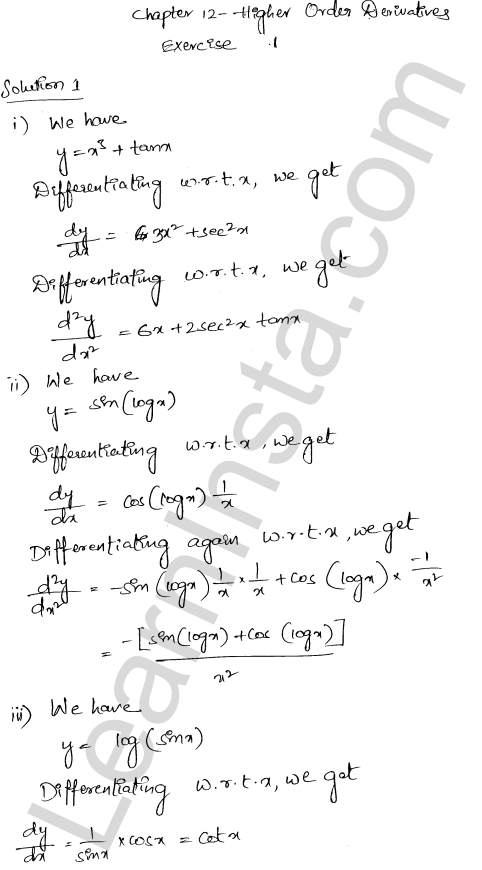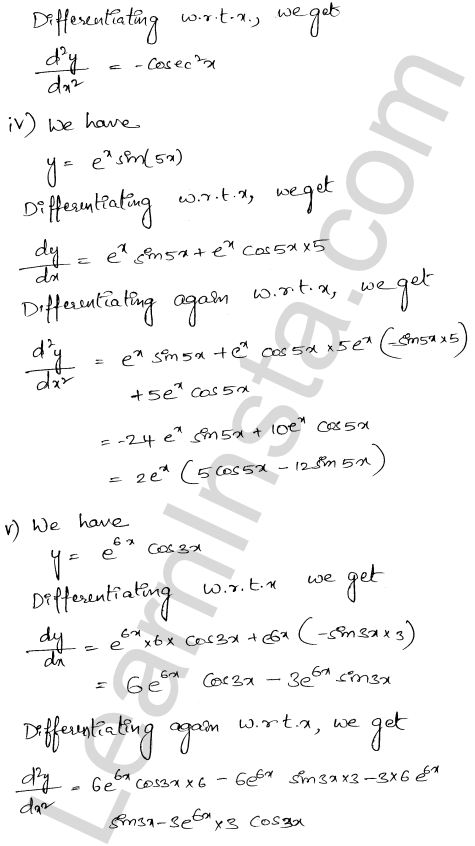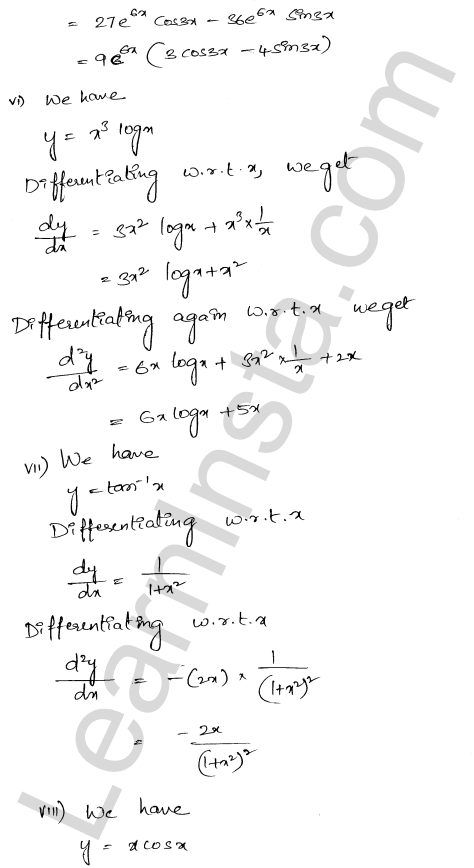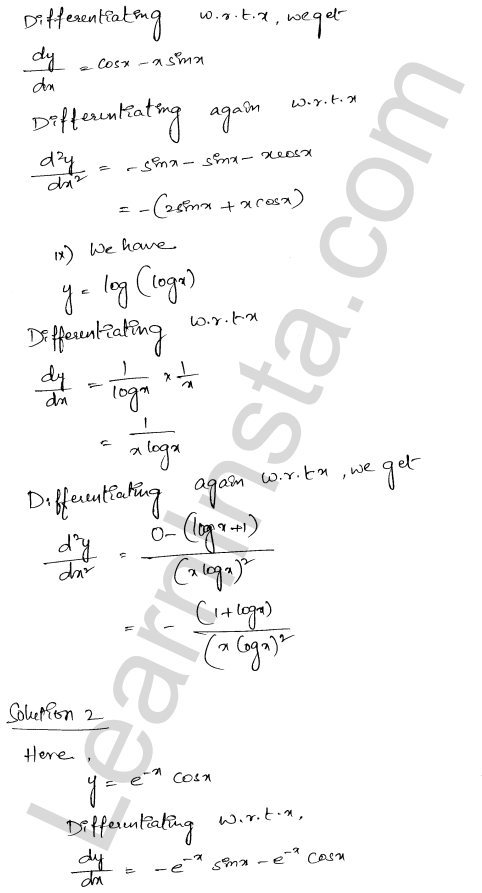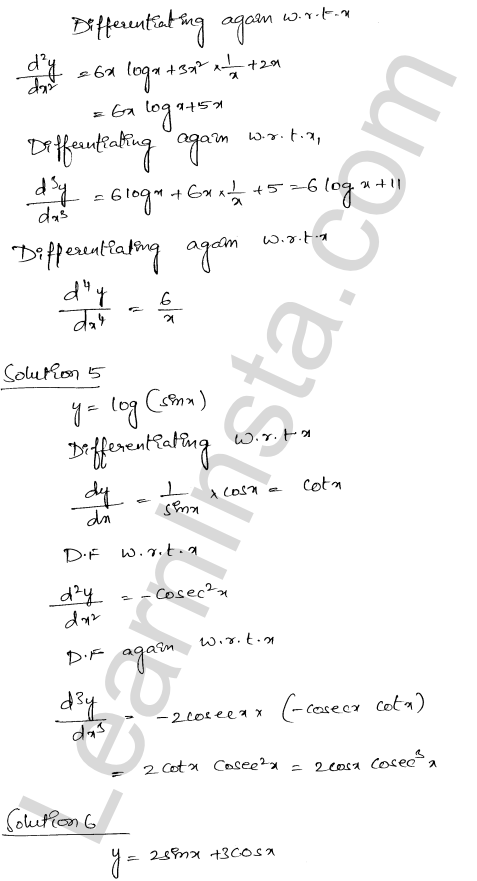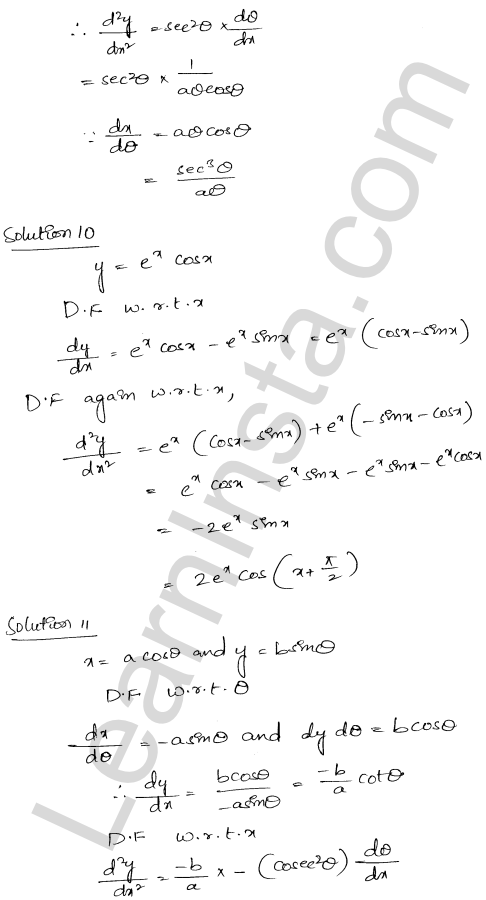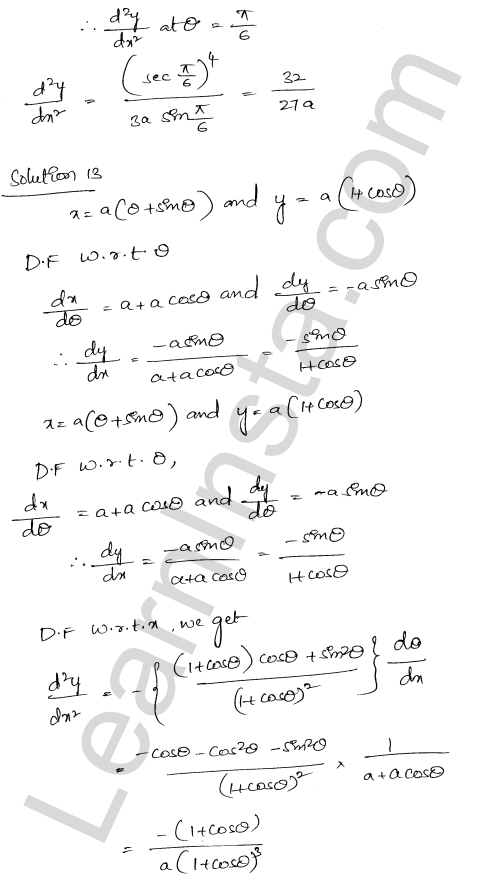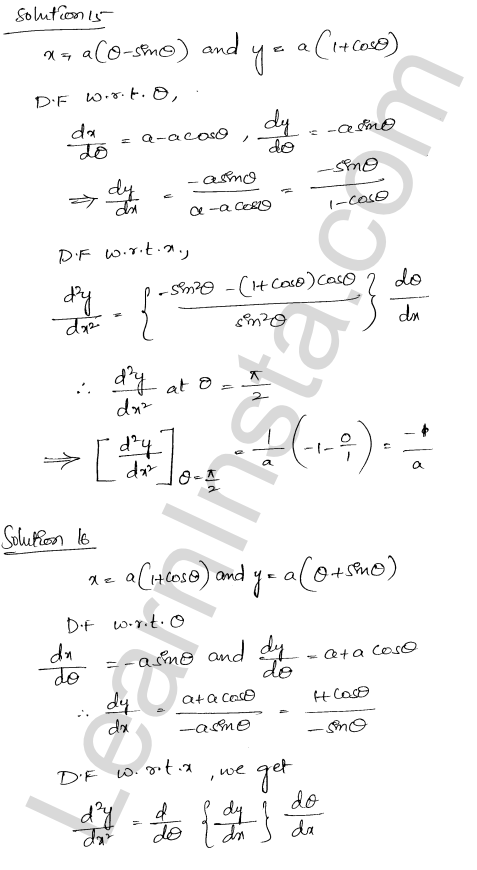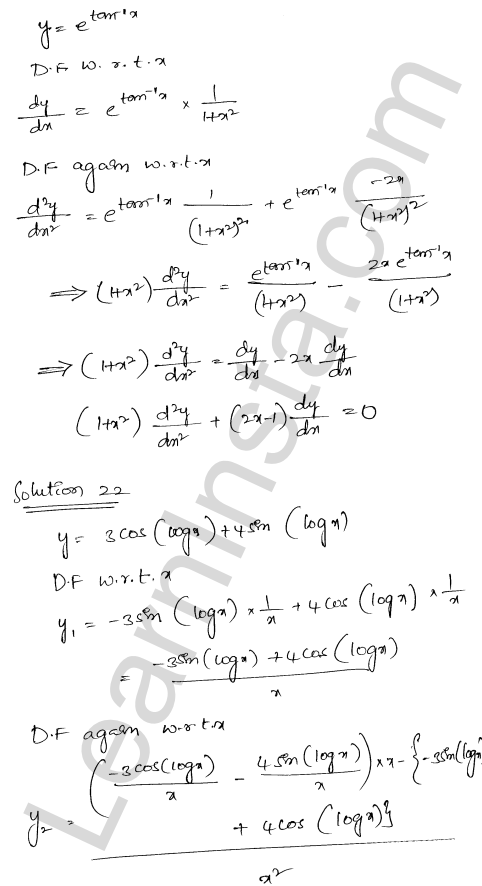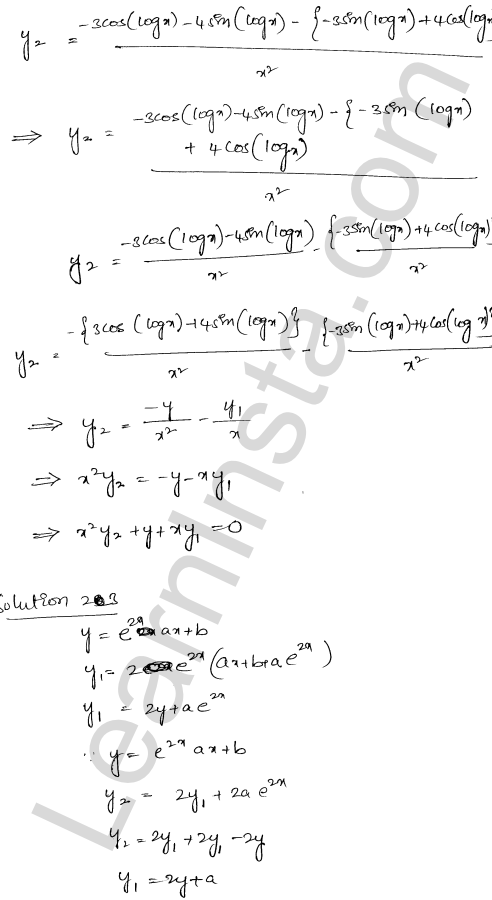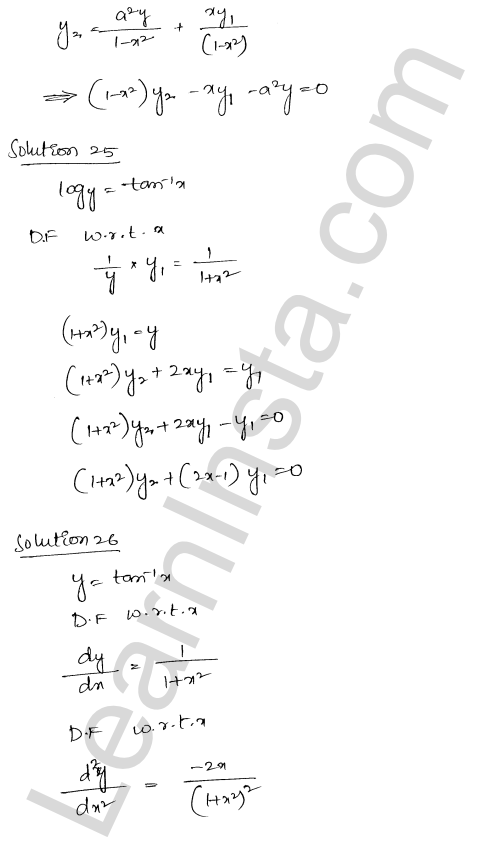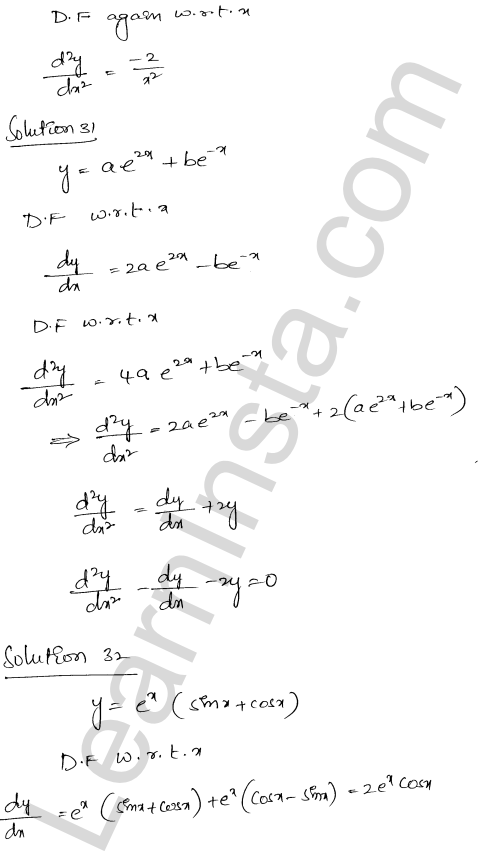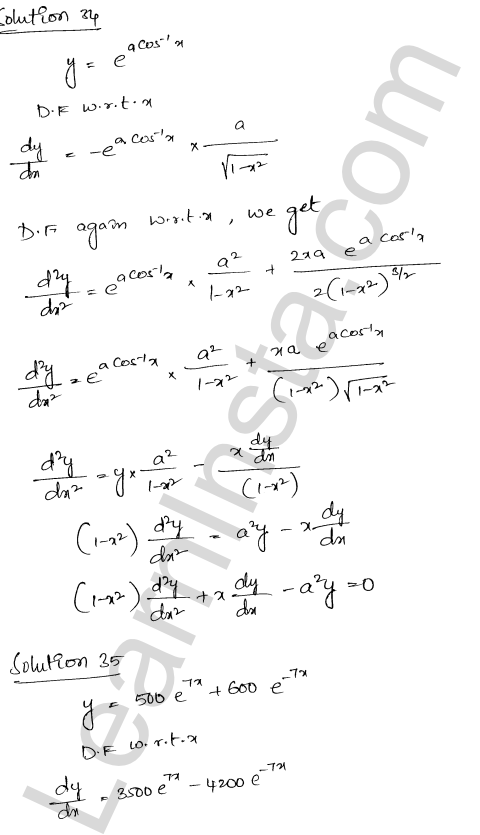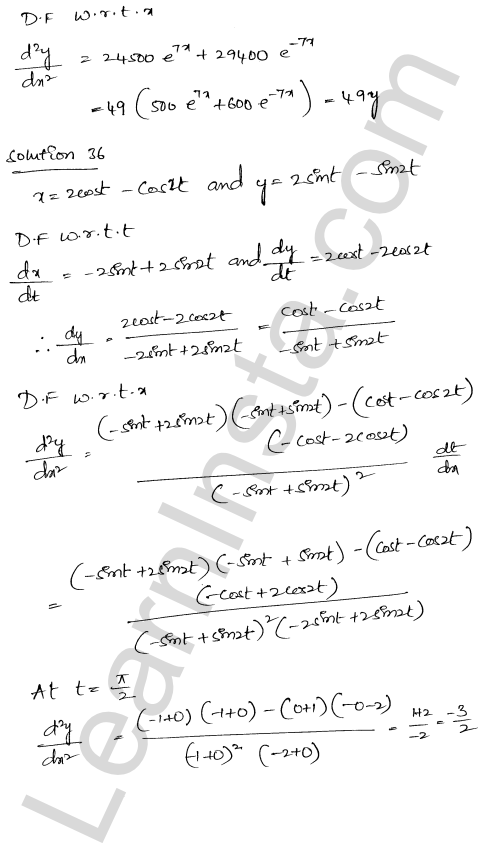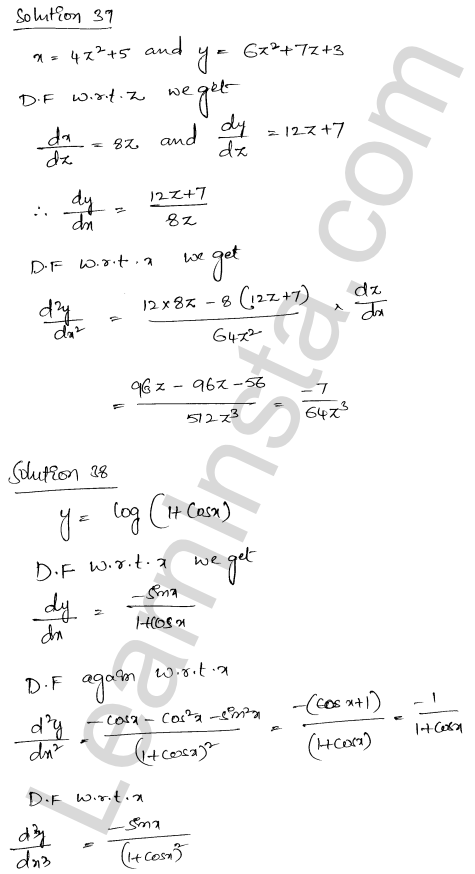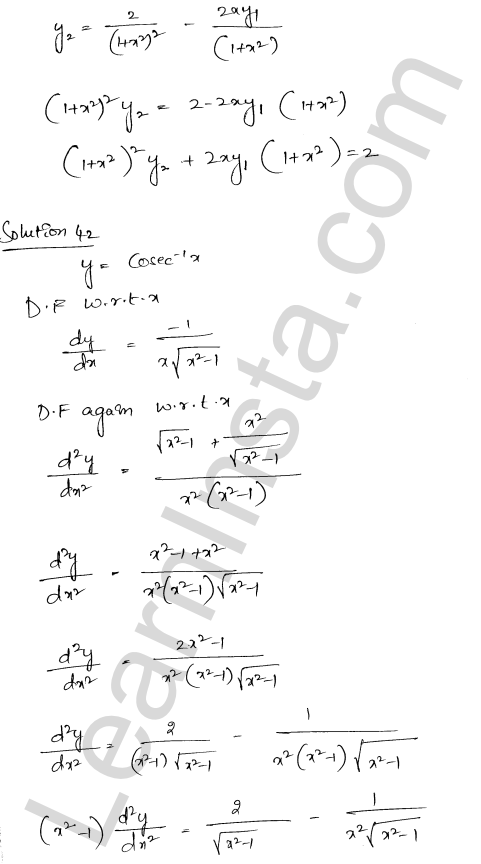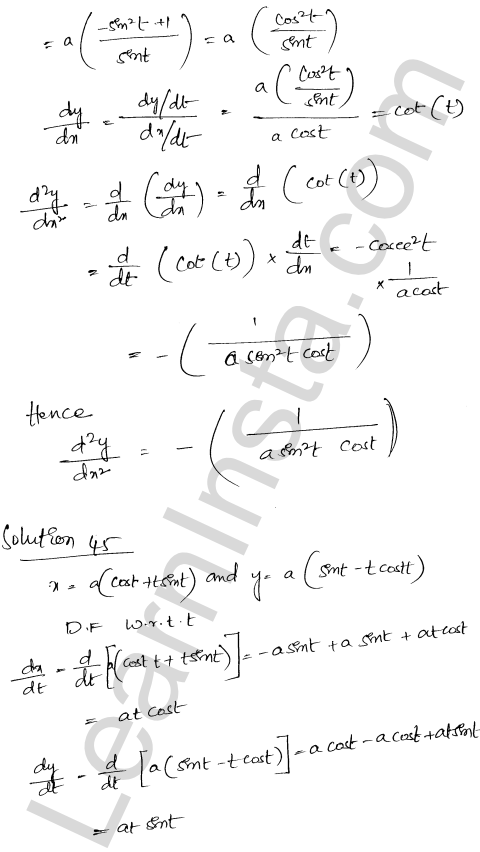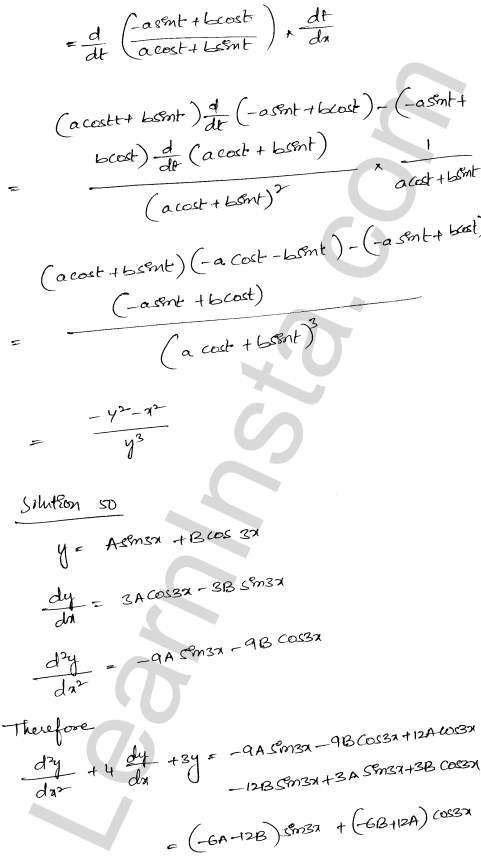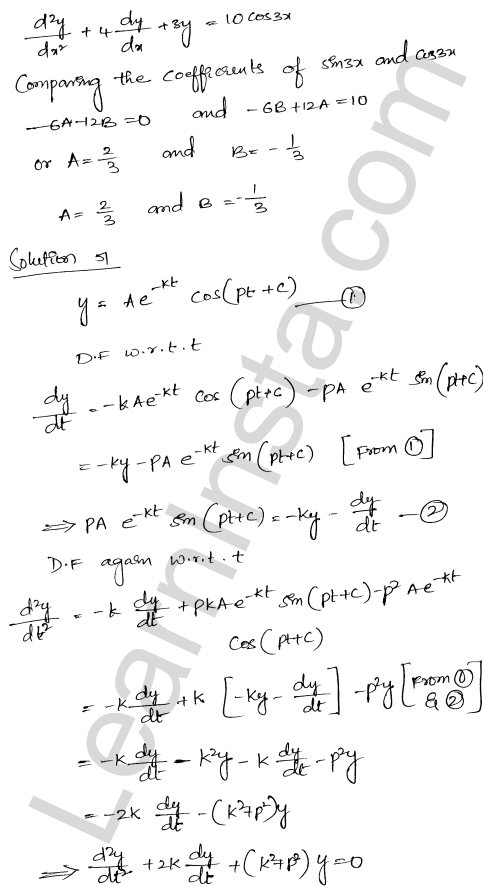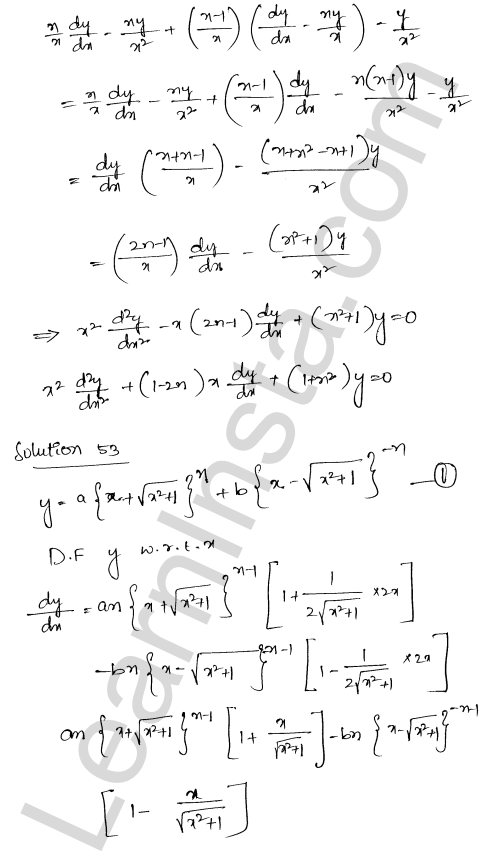We hope the RD Sharma Class 12 Solutions Chapter 12 Higher Order Derivatives Ex 12.1, help you. If you have any query regarding RD Sharma Class 12 Solutions Chapter 12 Higher Order Derivatives Ex 12.1, drop a comment below and we will get back to you at the earliest.